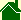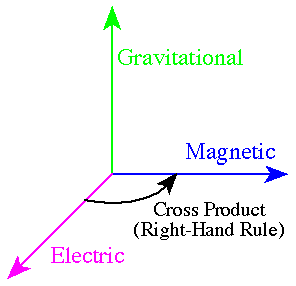#Summary of the Grand Unification Theory -- "The Ball-of-Light Particle Model"

### Nordberg Interpretation

Elementary particles are made up of three fields: electric, magnetic, and gravitational. These fields act at right angles to each other.The simple explanation is: the electric field cross the magnetic field equals the gravitational field.

The not-so-simple explanation is: a changing electric field induces a magnetic field; a changing magnetic field induces an electric field; the cross product of a changing electric field and a changing magnetic field induces a gravitational field; and a changing gravitational field induces an electromagnetic field. (See also Cepheid Variable.)

If any of the changing fields -- electric, magnetic, or gravitational -- have a great enough magnitude, then they can induce new particles of matter.

These 3 fields take on two natural states: standing waves and moving waves. When moving, the fields create a particle known as the photon.

These moving waves can collide. If the orientation and magnitude of the waves are correct -- see polarization -- then they can combine to create harmonic standing waves. (See also Supernova SN1987A)

The natural geometry of these waves is spherical. Therefore, elementary particles can be described as harmonic standing spherical waves of electric, magnetic and gravitational fields.

The natural "position" or "location" of the fields places the electric and magnetic pointing tangentially to the surface of the sphere. (This is the real meaning of "c2" in E = mc2.)

Thus, the cross product -- the gravitational field -- always points toward the center of the sphere. When this cross product is integrated over the surface of the sphere -- and using an, as yet, unnamed constant -- the result is the mass of the Ball-of-Light.

It is well known that particles of "matter" can not be accelerated to the speed of light. (See: As the bubble bursts velocity line.) The reason is: on the surface of a standing spherical wave of light are patches of electric and magnetic fields; these fields can have a motion around the sphere; if you accelerate a ball-of-light, then the motion of the sphere combines with the motion of the patches of electric and magnetic.

The first implication of this is, the motions can not add up to be greater than speed of light.

Second, accelerating these patches of electric and magnetic fields induce greater magnitudes in the electric and magnetic fields.

Third, increasing the magnitude of the electric and magnetic fields will increase the magnitude of the gravitational field.

Fourth, in general, up to a point, a higher gravitational field will make the particle more stable and contract it to a smaller size.

Finally, if the ball-of-light has asymmetrical patches of electric and magnetic to begin with, then depending on the nature of the asymmetries, accelerating the particle can: either make the particle less stable (the patches "pinch" the particle in half); or accelerating the particle can increase the gravitation field making the particle more stable.

(I believe all classical laws of physics will be directly derived from this explanation of the electromagnetic and gravitational induction.)

Scientists currently describe physics using the "Strong" and "Weak" nuclear forces. The Ball-of-Light Particle Model describes the strong force in terms of the natural attraction of two spherical waves of electric, magnetic, and gravitational fields. Just as the opposite poles of a magnet attract each other, and just as opposite electric fields attract each other, and just as gravitational field attract each other, the combination of: spherical electric, magnetic and gravitational fields -- with the correct geometry -- will attract even more. Obviously, a standing where all three known forces attract each other with perfect symmetry would outwardly appear to be the strongest force (i.e., the "Strong" force). (In retrospect, it is also easy to see why previously, this strong force might be thought of as one force, rather than as 3 combined forces.)

Sometimes, these three fields combine in combinations that are barely harmonic, somewhat harmonic, fairly harmonic, or completely harmonic. If the standing wave is not harmonic, then there is an asymmetrical electrical or magnetic force on the surface of the sphere that will cause the particle to decay. Currently, scientists interpret one of the situations of natural decay as the "Weak" Force. The Ball-of-Light Particle Model interprets the Weak Force as an asymmetry on the surface of a ball-of-light. This asymmetry accelerates -- inducing itself -- until it splits the particle in two.

This Grand Unification Theory does not set a limit on the size of an elementary particle. It could be the size: of a photon, a neutrino, an electron, a proton, a basketball (see Ball Lightning), a planet (See baby stars), the core of a star, the core of a galaxy, or even bigger. This theory predicts the Big Bang started as the decay of one single, very large, ball-of-light.

When thinking of the Ball-of-Light Particle Model, just remember Einstein's famous equation, E = mc2. Matter can be converted into energy and energy can be converted into matter because matter is made of energy -- light spinning around itself in spherical standing waves of electric, magnetic and gravitational energy.

Scientists do not have a Grand Unification Theory that includes an explanation of Gravity.